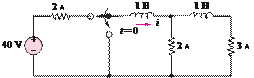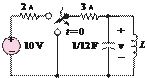### Create an Account

Already have account?

### Forgot Your Password ?

Home / Questions / For t 0 determine for what value of ti 6A in the circuit of Figure P578 if the circuit ...

# For t 0 determine for what value of ti 6A in the circuit of Figure P578 if the circuit is in steady state at t 0 and the maximum voltage between the contacts of the switch Figure

For 0determine for what value of ti = 6A in the circuit of Figure P5.78 if the circuit is in steady state at = 0−.

and the maximum voltage between the contacts of the switch.Figure P5.80

5.81 Find for 0 in the circuit of Figure P5.81 if the circuit is in steady state at = 0−.

Jun 18 2020 View more View Less

#### Answer (Solved)Subscribe To Get Solution